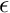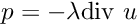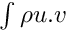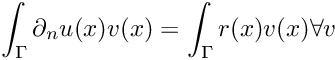GetFEM++  5.3
getfem_fourth_order.h File Reference

assembly procedures and bricks for fourth order pdes. More...

#include "getfem_models.h"
#include "getfem_assembling.h"

Go to the source code of this file.

## Namespaces

getfem
GEneric Tool for Finite Element Methods.

## Functions

template<typename MAT , typename VECT >
void getfem::asm_stiffness_matrix_for_bilaplacian (const MAT &M, const mesh_im &mim, const mesh_fem &mf, const mesh_fem &mf_data, const VECT &A, const mesh_region &rg=mesh_region::all_convexes())
assembly of.

size_type getfem::add_bilaplacian_brick (model &md, const mesh_im &mim, const std::string &varname, const std::string &dataname, size_type region=size_type(-1))
Adds a bilaplacian brick on the variable varname and on the mesh region region. More...

size_type getfem::add_bilaplacian_brick_KL (model &md, const mesh_im &mim, const std::string &varname, const std::string &dataname1, const std::string &dataname2, size_type region=size_type(-1))
Adds a bilaplacian brick on the variable varname and on the mesh region region. More...

template<typename VECT1 , typename VECT2 >
void getfem::asm_normal_derivative_source_term (VECT1 &B, const mesh_im &mim, const mesh_fem &mf, const mesh_fem &mf_data, const VECT2 &F, const mesh_region &rg)
assembly of.

size_type getfem::add_normal_derivative_source_term_brick (model &md, const mesh_im &mim, const std::string &varname, const std::string &dataname, size_type region)
Adds a normal derivative source term brick :math:F = b. More...

size_type getfem::add_Kirchhoff_Love_Neumann_term_brick (model &md, const mesh_im &mim, const std::string &varname, const std::string &dataname1, const std::string &dataname2, size_type region)
Adds a Neumann term brick for Kirchhoff-Love model on the variable varname and the mesh region region. More...

template<typename MAT , typename VECT1 , typename VECT2 >
void getfem::asm_normal_derivative_dirichlet_constraints (MAT &H, VECT1 &R, const mesh_im &mim, const mesh_fem &mf_u, const mesh_fem &mf_mult, const mesh_fem &mf_r, const VECT2 &r_data, const mesh_region &rg, bool R_must_be_derivated, int version)
Assembly of normal derivative Dirichlet constraintsin a weak form, whereis in the space of multipliers corresponding to mf_mult. More...

size_type getfem::add_normal_derivative_Dirichlet_condition_with_multipliers (model &md, const mesh_im &mim, const std::string &varname, const std::string &multname, size_type region, const std::string &dataname=std::string(), bool R_must_be_derivated=false)
Adds a Dirichlet condition on the normal derivative of the variable varname and on the mesh region region (which should be a boundary. More...

size_type getfem::add_normal_derivative_Dirichlet_condition_with_multipliers (model &md, const mesh_im &mim, const std::string &varname, const mesh_fem &mf_mult, size_type region, const std::string &dataname=std::string(), bool R_must_be_derivated=false)
Adds a Dirichlet condition on the normal derivative of the variable varname and on the mesh region region (which should be a boundary. More...

size_type getfem::add_normal_derivative_Dirichlet_condition_with_multipliers (model &md, const mesh_im &mim, const std::string &varname, dim_type degree, size_type region, const std::string &dataname=std::string(), bool R_must_be_derivated=false)
Adds a Dirichlet condition on the normal derivative of the variable varname and on the mesh region region (which should be a boundary. More...

size_type getfem::add_normal_derivative_Dirichlet_condition_with_penalization (model &md, const mesh_im &mim, const std::string &varname, scalar_type penalisation_coeff, size_type region, const std::string &dataname=std::string(), bool R_must_be_derivated=false)
Adds a Dirichlet condition on the normal derivative of the variable varname and on the mesh region region` (which should be a boundary. More...

## Detailed Description

assembly procedures and bricks for fourth order pdes.

Date
January 6, 2006.

Definition in file getfem_fourth_order.h.